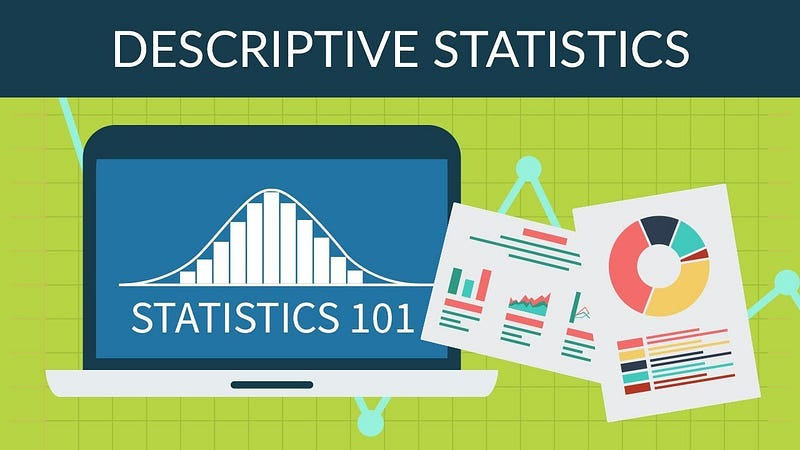# Mastering Descriptive and Inferential Statistics with R: A Comprehensive Beginner’s Guide### Introduction: The Foundations of Statistical Analysis with R

Statistics play a crucial role in data analysis, providing the tools and techniques to summarize, visualize, and draw insights from complex datasets. Descriptive and inferential statistics are two fundamental branches of statistical analysis, each serving distinct purposes in the data analysis process. In this comprehensive article, we will provide an in-depth guide to understanding and applying descriptive and inferential statistics using the R programming language, empowering beginners to tackle a wide range of data-driven challenges.

### 1. Descriptive Statistics: Summarizing and Visualizing Data

Descriptive statistics provide a summary of the main features of a dataset, helping analysts understand its structure, distribution, and relationships. Key descriptive statistics techniques include measures of central tendency, dispersion, and visualization methods.

#### 1.1 Measures of Central Tendency

Measures of central tendency describe the central point or average value of a dataset. The most common measures of central tendency are the mean, median, and mode.

– Mean: The arithmetic average of the dataset.
– Median: The middle value of the dataset when sorted in ascending order.
– Mode: The most frequently occurring value in the dataset.

In R, you can calculate these measures using the following functions:

```
mean(data\$column)
median(data\$column)
table(data\$column) # To find the mode, look for the highest count

```

#### 1.2 Measures of Dispersion

Measures of dispersion describe the spread or variability of a dataset. Common measures of dispersion include range, variance, and standard deviation.

– Range: The difference between the maximum and minimum values of the dataset.
– Variance: The average of the squared differences from the mean.
– Standard Deviation: The square root of the variance.

In R, you can calculate these measures using the following functions:

```range(data\$column)
var(data\$column)
sd(data\$column)```

#### 1.3 Data Visualization

Data visualization techniques, such as histograms, box plots, and scatter plots, can help analysts explore the distribution and relationships within a dataset.

– Histogram: A graphical representation of the distribution of a dataset using bars.
– Box Plot: A graphical representation of the distribution of a dataset using quartiles and whiskers.
– Scatter Plot: A graphical representation of the relationship between two variables using points.

In R, you can create these visualizations using the following functions:

```
hist(data\$column)
boxplot(data\$column)
plot(data\$column1, data\$column2)

```

### 2. Inferential Statistics: Drawing Conclusions from Data

Inferential statistics enable analysts to make inferences and predictions about populations based on samples. Key inferential statistics techniques include hypothesis testing, confidence intervals, and regression analysis.

#### 2.1 Hypothesis Testing

Hypothesis testing is a method for testing the validity of a claim or hypothesis about a population parameter based on a sample. The most common hypothesis tests are the t-test and the chi-square test.

– T-Test: Compares the means of two groups to determine if there is a significant difference between them.
– Chi-Square Test: Tests the relationship between categorical variables to determine if they are independent.

In R, you can perform hypothesis tests using the following functions:

```
t.test(data\$column1, data\$column2)
chisq.test(data\$column1, data\$column2)

```

#### 2.2 Confidence Intervals

A confidence interval provides an estimate of the range within which a population parameter is likely to fall, based on a sample. Confidence intervals can be calculated for means, proportions, and other population parameters.

In R, you can calculate confidence intervals using the following functions:

```
# For means
mean_ci <- function(data, conf_level = 0.95) {
n <- length(data)
mean_val <- mean(data)
se <- sd(data) / sqrt(n)
error_margin <- qnorm((1 + conf_level) / 2) * se
return(c(mean_val — error_margin, mean_val + error_margin))
}```
`mean_ci(data\$column)`
```# For proportions
prop.test(x = successes, n = trials, conf.level = 0.95)```

#### 2.3 Regression Analysis

Regression analysis is a statistical technique used to model the relationship between a dependent variable and one or more independent variables. The most common types of regression analysis are linear regression and logistic regression.

– Linear Regression: Models the relationship between a continuous dependent variable and one or more independent variables.
– Logistic Regression: Models the relationship between a binary dependent variable and one or more independent variables.

In R, you can perform regression analysis using the following functions:

```# Linear Regression
linear_model <- lm(dependent_variable ~ independent_variable, data = data)
summary(linear_model)```
```# Logistic Regression
logistic_model <- glm(dependent_variable ~ independent_variable, data = data, family = “binomial”)
summary(logistic_model)```

### Conclusion: Mastering Descriptive and Inferential Statistics with R

Understanding and applying descriptive and inferential statistics is essential for anyone looking to excel in data analysis. By mastering these techniques with R, beginners can develop a strong foundation in statistical analysis, empowering them to tackle complex data-driven problems and make informed decisions based on data. With a comprehensive understanding of measures of central tendency, dispersion, data visualization, hypothesis testing, confidence intervals, and regression analysis, beginners can confidently navigate the world of data analysis and derive valuable insights from their data.

# Personal Career & Learning Guide for Data Analyst, Data Engineer and Data Scientist

## Applied Machine Learning & Data Science Projects and Coding Recipes for Beginners

A list of FREE programming examples together with eTutorials & eBooks @ SETScholars

# Projects and Coding Recipes, eTutorials and eBooks: The best All-in-One resources for Data Analyst, Data Scientist, Machine Learning Engineer and Software Developer

Topics included:Classification, Clustering, Regression, Forecasting, Algorithms, Data Structures, Data Analytics & Data Science, Deep Learning, Machine Learning, Programming Languages and Software Tools & Packages.
(Discount is valid for limited time only)## Find more … …

How to plot Descriptive Statistics in R

Year Seven Math Worksheet for Kids – Applying Concept of Inferential Statistics

Statistics for Beginners with Excel – Descriptive Statistics Tools